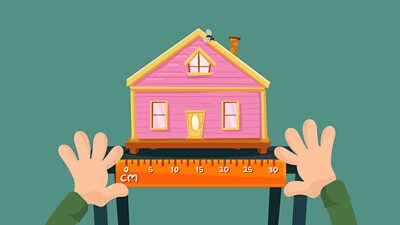# What are metric measurements?

The metric system is used to measure the length, weight or volume of an object. Length is measured in millimetres (mm), centimetres (cm), metres (m) or kilometres (km).

• 1 cm = 10 mm
• 1 m = 100 cm
• 1 km = 1000 m
• 1 cm is about the width of a staple
• 1 m is about the width of a single bed

Weight is measured in grams (g) and kilograms (kg). Volume is measured in millilitres (ml) and litres (l).

• 1 kg = 1000 g
• 1 l = 1000 ml
• 1 kg is the weight of seven apples
• 1 l is the volume of a carton of orange juice

## There's more to learn...

This guide